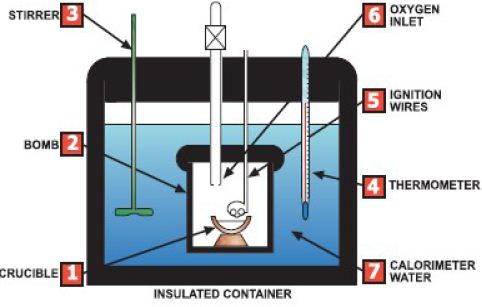Chemistry

# Measuring Enthalpy Changes by Calorimeter

A calorimeter is a device used to measure the heat absorbed or released during a physical or chemical change. Everything inside the bomb is the system. The water is the surroundings. The sample is ignited, and the heat absorbed by the calorimeter and its contents is the negative of the heat of reaction. A calorimeter is represented in the figure below.Measuring the Enthalpy change

Allow the reaction to heat (or cool) a known mass of water, measure the temperature change of the water then calculate the energy required using the formula; ΔH = – c m AT

Where:

• ΔH = the enthalpy change for the reaction,
• ΔT = the temperature change (in C or K) (the final temperature — initial temperature),
• m = mass of water (in kg), and
• c = specific heat capacity of water (4.18kJK-1.kg-1).

Example

0.253g of ethanol is burned in a calorimeter. The temperature of the surrounding 150g of water rises 10K. Calculate the enthalpy of combustion of ethanol.

Heat absorbed by water = c m ΔT = (4.18 x 0.15 x 10) kJ = 6.27 kJ

Molar mass of ethanol = 46g therefore moles of ethanol used = 0.253/46 = 0.0055 moles

Assuming all the heat produced by the combustion was absorbed by the water: 0.0055 moles of ethanol burns to give 6.2714 of heat. Therefore 1 mole of ethanol burns to give (6.27 x 1/0.0055) kJ = 1140 kJ of heat.